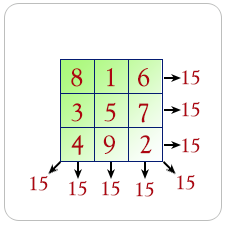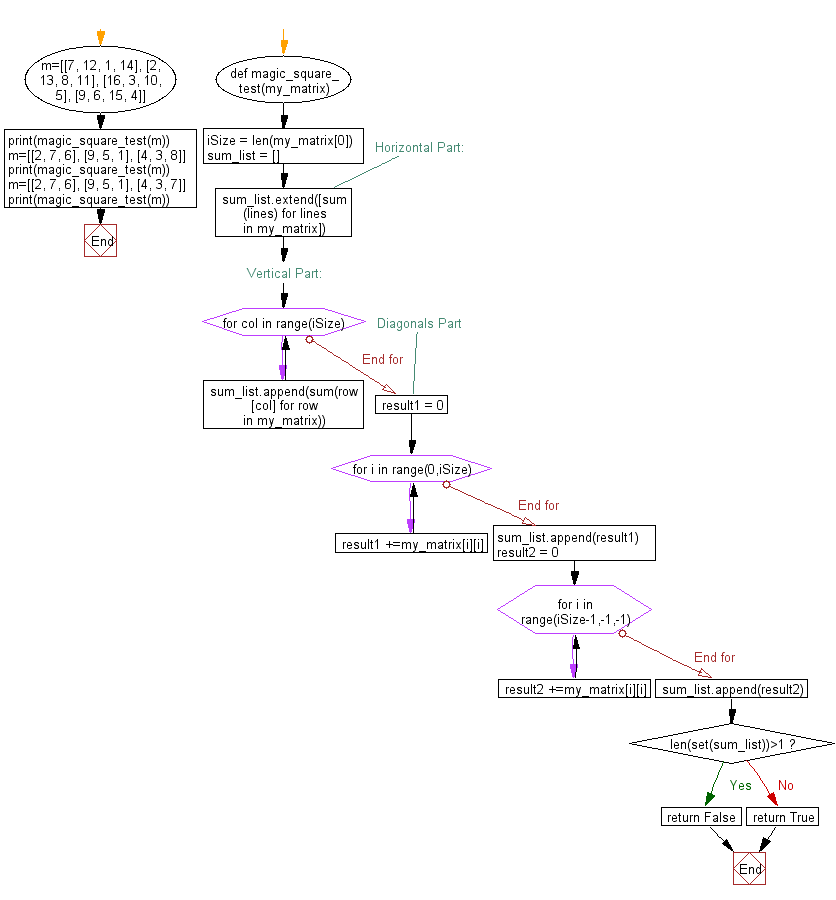﻿ Python Math: Calculate magic square - w3resource# Python Math: Calculate magic square

## Python Math: Exercise-20 with Solution

Write a Python program to calculate magic square.

A magic square is an arrangement of distinct numbers (i.e., each number is used once), usually integers, in a square grid, where the numbers in each row, and in each column, and the numbers in the main and secondary diagonals, all add up to the same number, called the "magic constant." A magic square has the same number of rows as it has columns, and in conventional math notation, "n" stands for the number of rows (and columns) it has. Thus, a magic square always contains n2 numbers, and its size (the number of rows [and columns] it has) is described as being "of order n".

Pictorial presentation:Sample Solution:-

Python Code:

``````def magic_square_test(my_matrix):
iSize = len(my_matrix)
sum_list = []

#Horizontal Part:
sum_list.extend([sum (lines) for lines in my_matrix])

#Vertical Part:
for col in range(iSize):
sum_list.append(sum(row[col] for row in my_matrix))

#Diagonals Part
result1 = 0
for i in range(0,iSize):
result1 +=my_matrix[i][i]
sum_list.append(result1)

result2 = 0
for i in range(iSize-1,-1,-1):
result2 +=my_matrix[i][i]
sum_list.append(result2)

if len(set(sum_list))>1:
return False
return True

m=[[7, 12, 1, 14], [2, 13, 8, 11], [16, 3, 10, 5], [9, 6, 15, 4]]
print(magic_square_test(m));

m=[[2, 7, 6], [9, 5, 1], [4, 3, 8]]
print(magic_square_test(m));

m=[[2, 7, 6], [9, 5, 1], [4, 3, 7]]
print(magic_square_test(m));
```
```

Sample Output:

```True
True
False
```

Flowchart:## Visualize Python code execution:

The following tool visualize what the computer is doing step-by-step as it executes the said program:

Python Code Editor:

Have another way to solve this solution? Contribute your code (and comments) through Disqus.

What is the difficulty level of this exercise?

Test your Programming skills with w3resource's quiz.

﻿

```>>> students = [{'name': 'John', 'score': 98}, {'name': 'Mike', 'score': 94}, {'name': 'Jennifer', 'score': 99}]